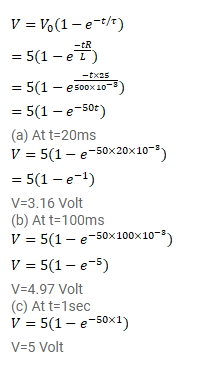# An LR circuit contains an inductor of

Question:

An LR circuit contains an inductor of $500 \mathrm{mH}$, a resistor of $25.0 \Omega$ and an emf of $5.00 \mathrm{~V}$ in series. Find the potential difference across the resistor at

(a) $t=$ $20.0 \mathrm{~ms}$,

(b) $100 \mathrm{~ms}$ and

(c) $1.00 \mathrm{~s}$.

Solution: